## 7.7Turbulence near walls

The characteristic velocity distribution in turbulent boundary layers in Sec. 7.4 , provided wall functions expressed as boundary conditions for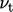in Sec. 7.5 and Sec. 7.6 . Boundary conditions also need to be speciﬁed for turbulence ﬁelds at solid walls.

The turbulence generation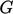inﬂuences the distribution of turbulence ﬁelds near a wall. At the wall,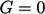. In the inertial sub-layer,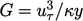from Eq. (7.5 ) with: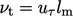, obtained by combining Eq. (6.21 ) and Eq. (7.15 ); and, Eq. (6.24 ).

Sincedecreases with increasing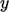in the inertial sub-layer,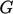passes through a peak within the buﬀer layer (at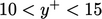).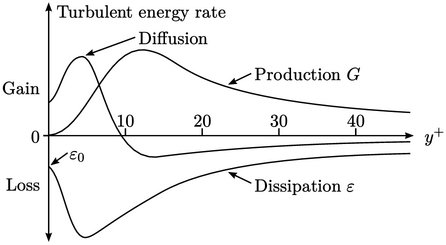The peak incauses a similar peak in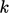, shown in the following diagram. To the left of the peak, turbulent energy is transported back towards the wall by diﬀusion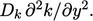The proﬁle of dissipation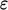results from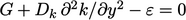, obtained from Eq. (7.1 ). Very close to the wall (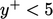), diﬀusion is predominately molecular, such that it non-zero at the wall. The dissipation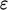also has a non-zero value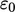at the wall.

### Wall functions and turbulence ﬁelds

When using a turbulence model, such as the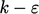model described in Sec. 7.1 , boundary conditions must be speciﬁed for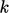andat solid walls. The distribution of, non-dimensionalised as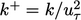, close to the wall is shown below.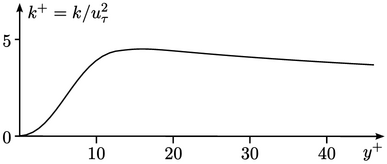At the wall,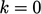but it rises quickly to a peak at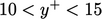before levelling oﬀ at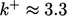as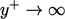.

With wall functions, the height of the centre of each near-wall cell should correspond to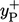within the range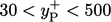. Viewed at that scale, the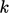proﬁle appears ﬂat. For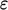, there is no such simple proﬁle shape. These observations lead to the boundary conditions forandwhen using wall functions:

• zero gradient for;
• calculated near-wall cell value for, according to: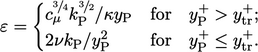(7.26)
The calculation uses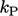from the near-wall cell. The expression for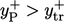is Eq. (7.4 ) with Eq. (6.24 ). The expression for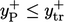uses the asymptotic condition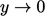for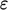in Eq. (7.28 ).
Notes on CFD: General Principles - 7.7 Turbulence near walls